# Understanding Collision Constraint Solvers

### Published on Sunday, March 21st, 2021

Constraints are a useful way to build relatively cheap, stable, and efficient physics systems. Lots of cool stuff can be unlocked with this approach 😎

In this post I want to help people understand constraints too!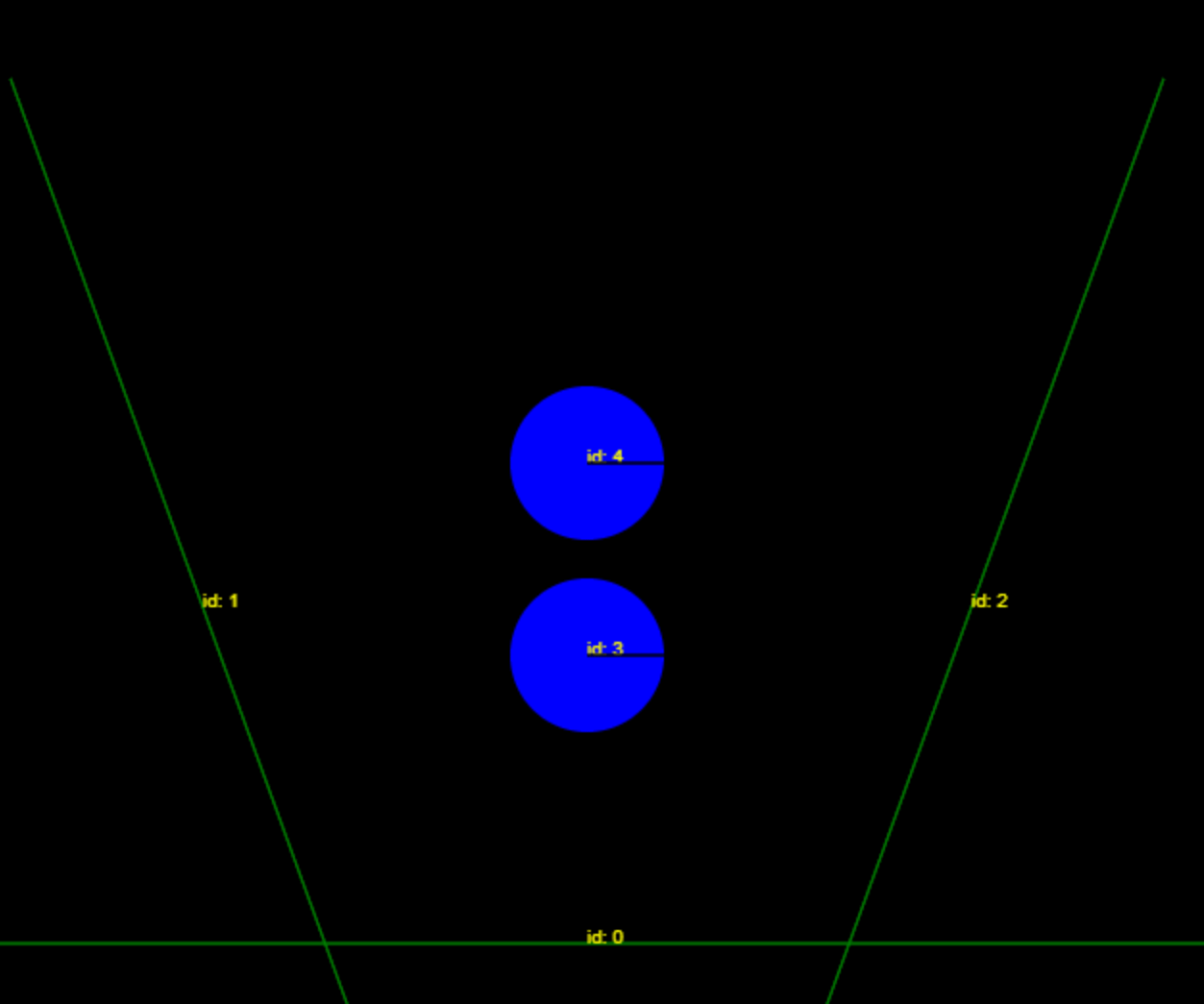I'll admit I had a hard time wrapping my brain 🧠 around using constraints to resolve collisions. I watched this amazing talk by Erin Catto at GDC 2014 and wanted to replicate and understand the technique.

What I want to do is reason out why these constraints might be the way they are by intuition and perhaps helpful ways to think about constraints.

The actual derivation is better served by Erin's talk, I recommend you check it out. I've probably watched it 10 times 😉

TLDR show me the code

### What is a "Constraint"

Think of a constraint like a statement you want to be true and the "solver" attempts to make it true. Examples of constraints in a physics system might be: "I don't want these two objects to overlap", "I want these two objects to be attached to another", or "I want these two objects to bounce off each other"

A constraint solver, takes all the contraints in your system and finds a solution that satisfies them all.

In theory a set of constraints can be thought of as a group of related equations that can be solved. A stack of colliding objects is a good example of related contraints where the solution is their correct positions and velocities without any overlap.

Awesome! I think I get it. How do I turn those into code?

### Solving with Sequential Impulse

In this setup we are going to attempt to "solve" these constraints by applying a series of "impulses" to each object in contact. Once enough impulses are applied over time, a stable configuration of all the objects under constraints should fall out the other side.

I have a goofy metaphor for this. Picture shaking a box of puzzle pieces that have magic magnets which are attracted to the right position. Shake until it's solved. 🤷‍♂️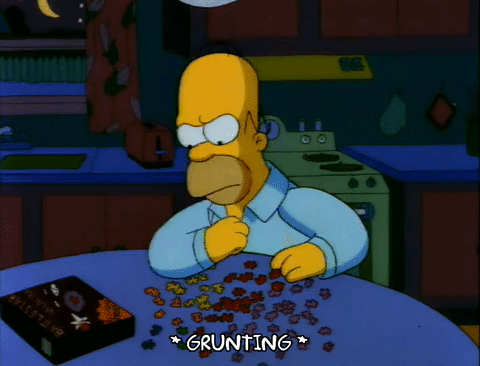Okay, what is an impulse? Well an impulse is defined as force added up over a time period. A super quick derivation from Newtonian physics from the familiar 2nd law:

`force = mass * acceleration`

Acceleration is another way of saying change in velocity in a given time step so re-written:

`force = mass * deltaVelocity/deltaTime`

Multiply by `deltaTime`

`force * deltaTime = mass * deltaVelocity`

Force over a time is the definition of impulse, so we can solve for our velocity. We will call our time period is the same for each frame so you can think of it as 1 unit of time 😎

`impulse = mass * deltaVelocity`

`deltaVelocity = impulse * (1/mass)` - Linear Impulse

The angular version of this has a similar formulation, but I'll cut to the chase.

`deltaAngularVelocity = impulse x (1/momentOfInertia)` - Angular Impulse

``/** * Apply an impulse at a point * @param point  * @param impulse  * @returns  */applyImpulse(point: Vector, impulse: Vector) {    if (this.static) {        return;    }    // Important for the torque term, the distance from the center    // Changes the rotational amount applied     const distanceFromCenter = point.sub(this.xf.pos);    this.m.vel = this.m.vel.add(impulse.scale(this.inverseMass));    this.m.angularVelocity += this.inverseInertia * distanceFromCenter.cross(impulse);}``

### Building Constraints - Overlap

Let's start with "not allowing objects to overlap." We know the exact amount that two circles overlap with each other. We'll say that a negative separation means overlap.

``let combinedRadius = circleA.radius + circleB.radiuslet distance = circleA.pos.distance(circleB.pos);if (distance < combinedRadius) {  let separation = combindedRadius - distance;  // making it negative to signify "overlap"  // only a convention for the math  return -separation;}``

To avoid overlap we want to trend `separation === 0` over time.

To do this, we can apply a steering factor every iteration to push `separation` towards 0. In the biz 😎, this steering factor is called Baumgarte stabilization, the idea of which is to feed the current "error", scaled by a chosen constant, back into the solver.

Erin Catto suggests not feeding the position correction term into the actual velocities because it introduces more artificial kinetic energy into the system, this differs from other solver implementations that do correct position overlap with velocity based impulses. Erin calls this positional approach a "pseudo-impulse".

``// DO THIS FOR X Iterations per frame, in practice only a couple are necessaryfor (let contact of contacts) {  for (let point of contact.points) {    const separation = contact.getSeparation();    // Magic number by 0.2 seems to be a good amount    const steeringConstant = 0.2    // Limit the amount of correction at once for stability    const maxCorrection = -5;    // Be tolerant of 1 pixel of overlap    const slop = 1;    // Clamp to avoid over-correction    // Remember that we are shooting for 0 overlap in the end    const steeringForce = clamp(steeringConstant * (separation + slop), maxCorrection, 0);    const impulse = contact.normal.scale(-steeringForce / point.normalMass);    // NOTE! Update positions of bodyA & bodyB directly by "pseudo-impulse", we still use the same impulse formula from above    if (!bodyA.static) {        bodyA.xf.pos = bodyA.xf.pos.add(impulse.negate().scale(bodyA.inverseMass));        bodyA.xf.rotation -= point.aToContact.cross(impulse) * bodyA.inverseInertia;    }    if (!bodyB.static) {        bodyB.xf.pos = bodyB.xf.pos.add(impulse.scale(bodyB.inverseMass));        bodyB.xf.rotation += point.bToContact.cross(impulse) * bodyB.inverseInertia;    }  }}``

In theory we could adjust both body's by the exact overlap (`steeringConstant === 1.0`), which we do know, but this introduces overlap in other shapes and the simulation ends up looking pretty terrible with shapes bouncing back and forth into each other.

Example of `steeringConstant === 1.0`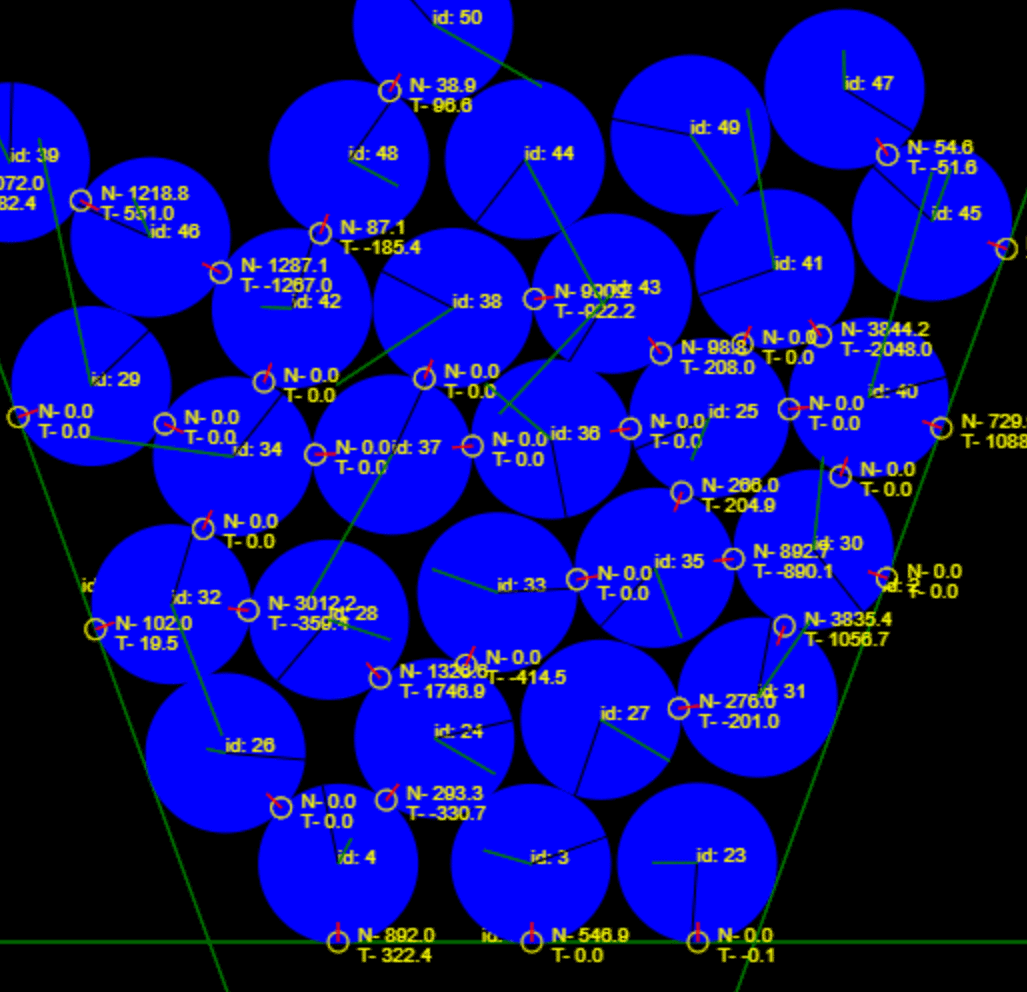### Build Constraints - Velocity Collision Response

To prevent overlap in the direction of collision, we want the relative velocity of the two shapes to be 0 along the normal. So `relativeVelocity.dot(normal) === 0`

In this code we calculate the velocity in the normal direction and apply the impulse in the opposite direction to cancel it out. Voila! Constraint met!

Hand-waving some of the Newtonian math, impulses works out like so for us. (More reading on impulses)

`effectiveMass * deltaVelocity = impulse`

Given this formula, if we can solve for an `impulse` that will yeild a `deltaVelocity` hat will bring velocity to 0 along the normal we are all set! See below:

``// DO THIS FOR X Iterations per frame, in practice 6ishfor (let contact of contacts) {  for (let point of contact.points) {    // Need to recalc relative velocity because the previous step could have changed vel    const relativeVelocity = point.getRelativeVelocity();    const effectiveMass = bodyA.inverseMass + bodyB.inverseMass +                           bodyA.inverseInertia * aToContactNormal * aToContactNormal +                          bodyB.inverseInertia * bToContactNormal * bToContactNormal;    // Compute impulse in normal direction    const normalVelocity = relativeVelocity.dot(contact.normal);    let impulseDelta = -normalVelocity / effectiveMass;    let impulseVector = contact.normal.scale(impulseDelta);        // By convention the normal points away from A, so negate    contact.bodyA.applyImpulse(point.point, impulseVector.negate());    contact.bodyB.applyImpulse(point.point, impulse);  }}``

This is only part of the picture the above only, what about objects bouncing off of each other? Borrowing from the wiki page for impulse based reactions the formula for this is:

`-(1 + restitution) * relativeVelocity.dot(normal) / effectiveMass`

Restitution is the ratio the body velocities after collision, one way to think about this is perhaps the "bounciness" of the system. Values of restitution should be between 0 and 1.

``// DO THIS FOR X Iterations per frame, in practice 6ishfor (let contact of contacts) {  for (let point of contact.points) {    // Need to recalc relative velocity because the previous step could have changed vel    const relativeVelocity = point.getRelativeVelocity();    const effectiveMass = bodyA.inverseMass + bodyB.inverseMass +                           bodyA.inverseInertia * aToContactNormal * aToContactNormal +                          bodyB.inverseInertia * bToContactNormal * bToContactNormal;    const restitution = bodyA.restitution * bodyB.restitution;    // Compute impulse in normal direction    const normalVelocity = relativeVelocity.dot(contact.normal);    let impulseDelta = (-(1 + restitution) * normalVelocity) / effectiveMass;    let impulseVector = contact.normal.scale(impulseDelta);        // By convention the normal points away from A, so negate    contact.bodyA.applyImpulse(point.point, impulseVector.negate());    contact.bodyB.applyImpulse(point.point, impulse);  }}``

Now the resulting velocity after is the opposite in the normal direction scaled according to the restitution term! Voila we have bouncing! ✨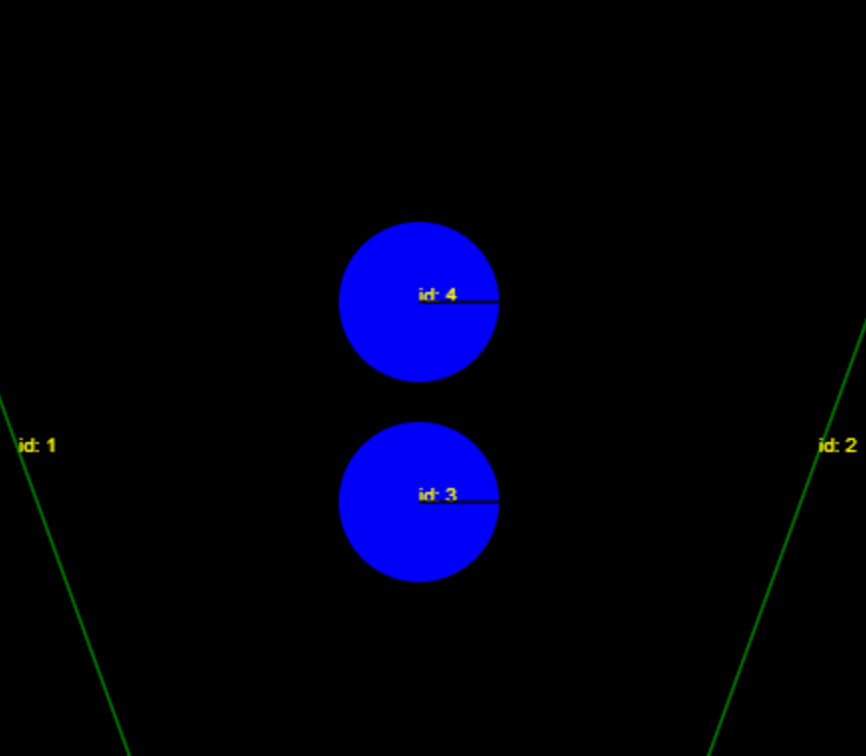### Build Constraints - Friction

For friction, the process is similar to the constraint in the normal direction. We want to trend velocity in the tangent direction `relativeVelocity.dot(tangent) === 0`

Notice that the effective mass in the tangent direction is different than the normal direction.

``for (let contact of contacts) {  for (let point of contact.points) {    const relativeVelocity = point.getRelativeVelocity();    const effectiveMass = bodyA.inverseMass + bodyB.inverseMass +                          bodyA.inverseInertia * aToContactTangent * aToContactTangent +                          bodyB.inverseInertia * bToContactTangent * bToContactTangent;    const tangentVelocity = -relativeVelocity.dot(contact.tangent);    let impulseDelta = tangentVelocity / point.tangentMass;    // Impulse along the TANGENT    let impulseVector = contact.tangent.scale(impulseDelta);        // By convention the normal points away from A, so negate    contact.bodyA.applyImpulse(point.point, impulseVector.negate());    contact.bodyB.applyImpulse(point.point, impulse);  }}``

Not too bad, and it produces a pretty believable simulation.

### Contact Warming for Stability

We can take advantage of previous work in earlier frames to help improve simulation stability (especially for large stacks of objects) by accumating the impulse for contacts that persist across frames. This helps overcome the force of gravity on big stacks by giving objects at the bottom extra impulse to start with.

Notice compression of the stack when warming is off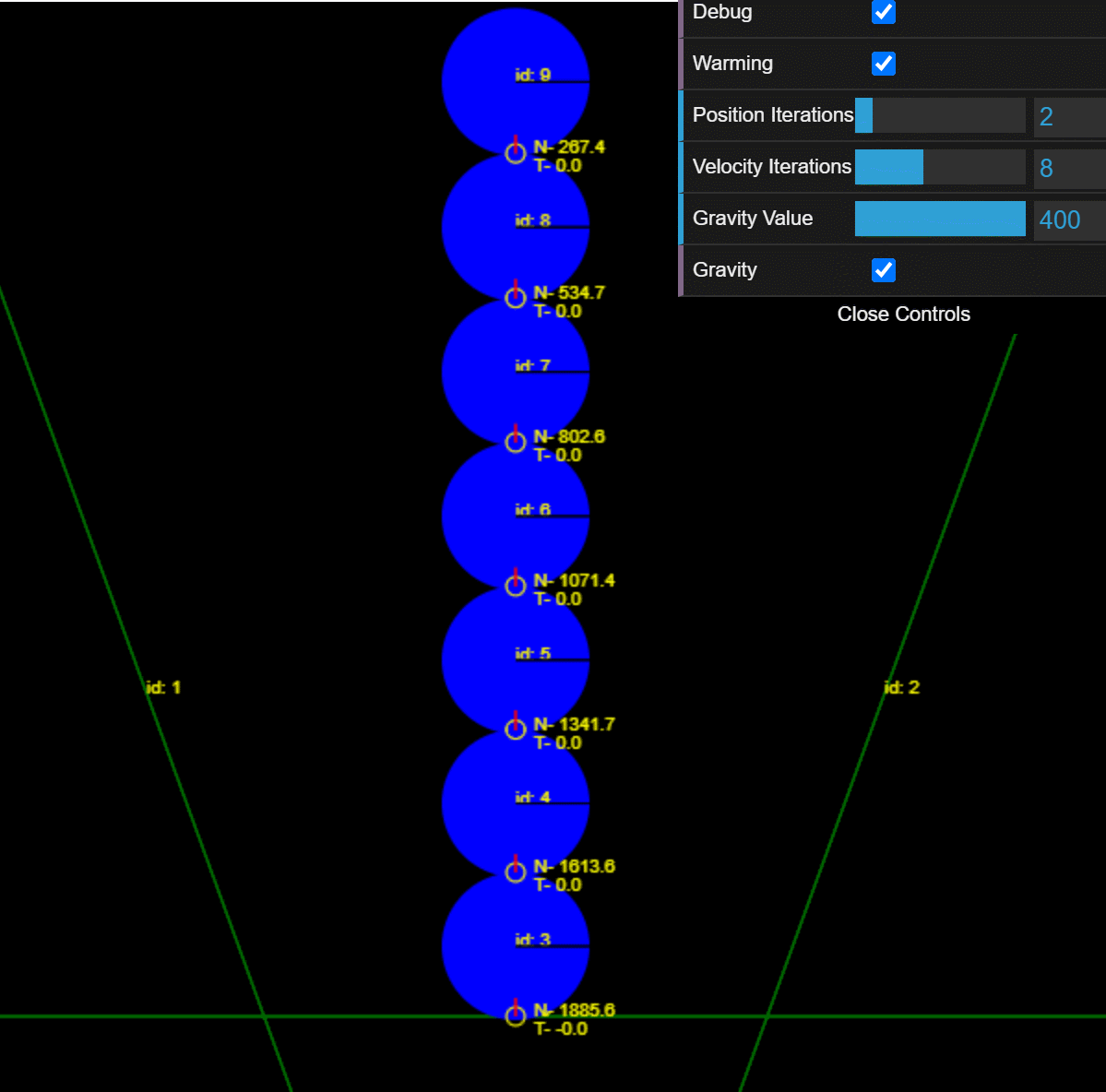There is a trick to this, you can't just stash all the the impulses. We need to preserve the constraint that the sum of all impulses is positive (`Velocity >= 0`) over time or it's possible shapes will end up overlaping on warming.

In these examples we keep track of accumulated impulses in each persistent contact point.

``// Clamping based in Erin Catto's GDC 2014 talk// Accumulated impulse stored in the contact is always positive (V >= 0)const newImpulse = Math.max(point.normalImpulse + impulseDelta, 0);// But current impulse deltas can be negativeimpulseDelta = newImpulse - point.normalImpulse;point.normalImpulse = newImpulse;``

Friction needs an additional check to keep things proportional to the normal force. Intiutively this makes sense, the harder something is pushing down on a surface, the more it sticks and resists sliding.

``// Clamping based in Erin Catto's GDC 2006 talk// Correct clamping https://github.com/erincatto/box2d-lite/blob/master/docs/GDC2006_Catto_Erin_PhysicsTutorial.pdf// Accumulated fiction impulse is always between -uMaxFriction < dT < uMaxFriction// But deltas can varyconst maxFriction = friction * point.normalImpulse;const newImpulse = clamp(point.tangentImpulse + impulseDelta, -maxFriction, maxFriction);impulseDelta = newImpulse - point.tangentImpulse;point.tangentImpulse = newImpulse;``

Once we have the impulses, at the beginning of the frame we can apply the accumulated impulses to any body's part of a persistent contact.

``for (let contact of contacts) {  for (let point of contact.points) {    const normalImpulse = contact.normal.scale(point.normalImpulse);    // Scaling back the tangent impulse seems to increase stack stability?    const tangentImpulse = contact.tangent.scale(point.tangentImpulse).scale(.2);    const impulse = normalImpulse.add(tangentImpulse);      contact.bodyA.applyLinearImpulse(normalImpulse.negate());    contact.bodyA.applyAngularImpulse(point.point, tangentImpulse.negate());    contact.bodyB.applyLinearImpulse(normalImpulse);    contact.bodyB.applyAngularImpulse(point.point, tangentImpulse);  }}``

### Order is important

Order of solving constraints creates precedence, constraints solved later in the frame take precedence. In general, your position constraints (like overlap) will probably take the most precedence in your simulation.

Lowest precedence

1. Warm Contacts
2. Friction
3. Collsion Response (Velocity)
4. No Overlap (Position)

Highest precedence

### Summary

Constraint based collision resolution feels very similar in principle to other steering approaches, like flocking AI's, or enemy/npm AI. Steering is a powerful technique to create interesting emergent behavior with simple rules.

Things to explore next with constraints might be joints, motors, etc using constraints!